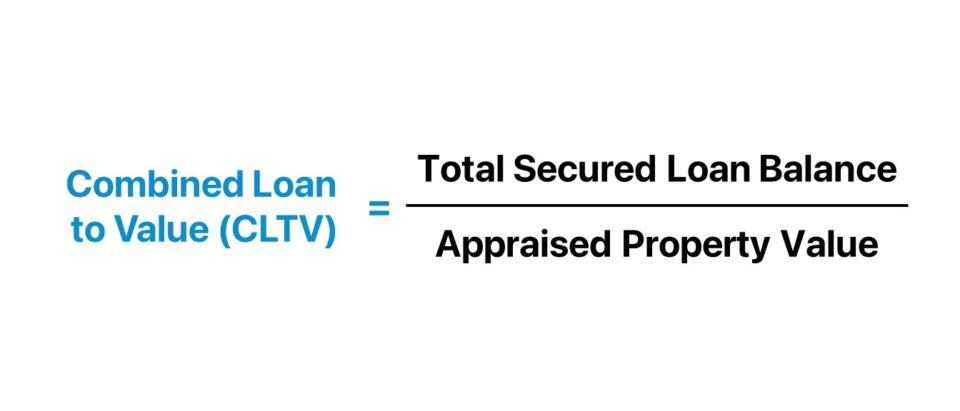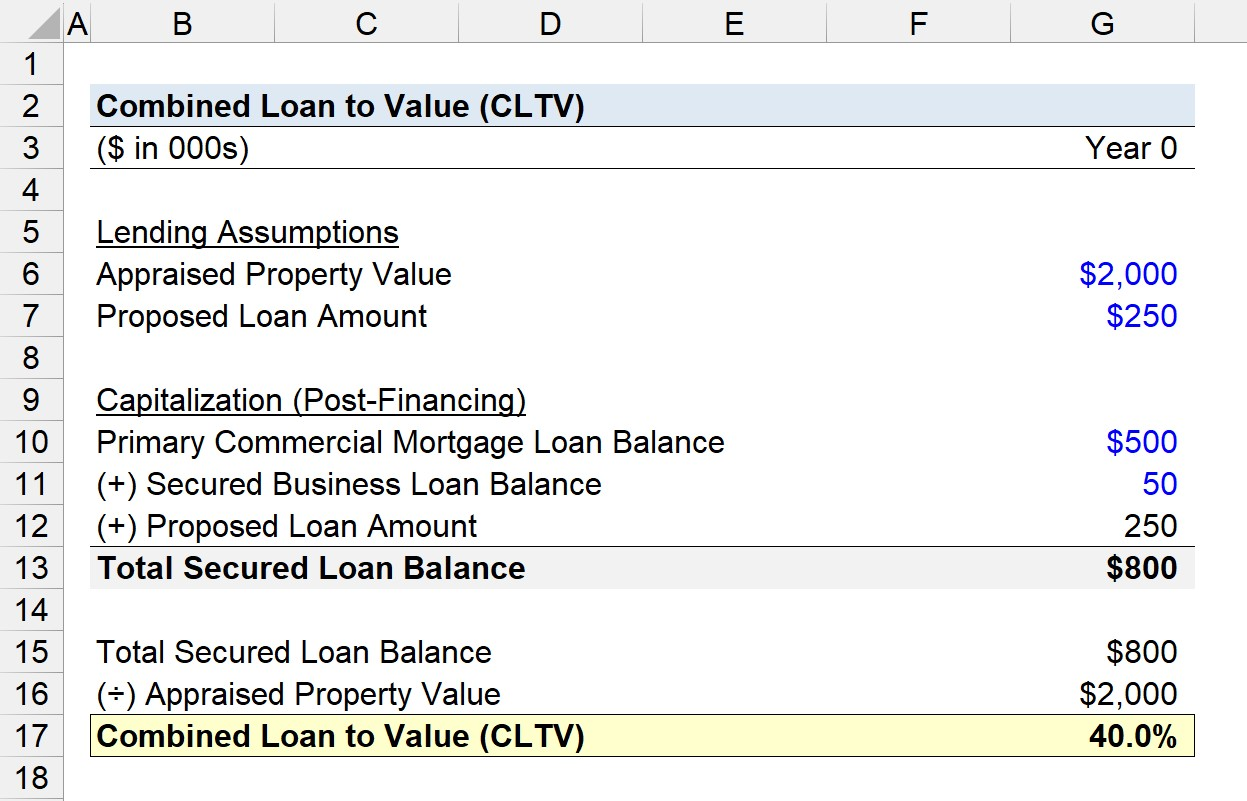Welcome to Wall Street Prep! Use code at checkout for 15% off.# Combined Loan to Value (CLTV)

Guide to Understanding Combined Loan to Value (CLTV)## How to Calculate Combined Loan to Value (CLTV)?

The combined loan to value, or CLTV ratio, is an underwriting metric used by real estate lenders to determine the risk of a proposed borrowing.

In particular, lenders use the combined loan to value ratio (CLTV) in scenarios where there is an existing lien against the underlying property asset, i.e. there is more than one secured form of financing.

The term “lien” is defined as a lender’s claim and right to seize possession of an asset belonging to the borrower until the entire debt obligation is fulfilled.

Since multiple secured loans cause the risk to all participants to rise, the CLTV ratio measures the default risk of the borrower, while factoring in the existing liens on the property.

Generally speaking, a lower CLTV ratio implies less risk for the lender, because there is a greater percentage of the property value not being borrowed against (and vice versa for a higher CLTV ratio).

The CLTV ratio is therefore intended to mitigate the lender’s downside risk potential and minimize the losses incurred in the event that the borrower defaults, i.e. misses an interest payment on a loan.

The process of calculating the combined loan to value ratio (CLTV) is as follows.

1. Calculate the Total Balance of Existing Secured Loans on the Property
2. Determine the Appraised Value of the Property, i.e. Current Fair Market Value (FMV)
3. Divide the Total Balance of Secured Loans by the Appraised Property Value## Combined Loan to Value Formula (CLTV)

The combined loan to value (CLTV) ratio is as follows.

Combined Loan to Value (CLTV) = Total Secured Loan Balance ÷ Appraised Property Value

Where:

• Total Secured Loan Value → The current outstanding balance of the secured loans (i.e. existing liens) on a given property.
• Appraised Property Value → The market value of the property as of the present date based on a formal appraisal.

To convert the CLTV ratio from decimal notation into percentage form, the output must be multiplied by 100.

For example, suppose a homeowner with a primary mortgage of \$200k is requesting a home equity loan of \$50k with a lien placed against the same property.

If the home is currently appraised at \$500k, the implied combined loan-to-value (CLTV) ratio is 50.0%.

• Combined Loan to Value Ratio (CLTV) = \$250k ÷ \$500k = .50, or 50.0%

## CLTV Ratio vs. LTV Ratio: What is the Difference?

The combined loan-to-value (CLTV) ratio is virtually identical to the loan-to-value (LTV) ratio, with the circumstances of the borrowing being the only notable distinction.

Like the loan-to-value ratio (LTV), the CLTV ratio describes the relationship between the loan amount and the appraised fair value of the property securing the loan.

Loan to Value Ratio (LTV) = Loan Amount ÷ Appraised Property Value

However, the loan-to-value ratio (LTV) only considers the initial or principal mortgage, whereas the CLTV ratio factors in the sum of all existing secured loans on a property.

If there is a pre-existing lien on the property, lenders use the CLTV ratio instead of the LTV ratio to estimate the risk of default.

While the maximum allowable threshold is contingent on the specific lender, most lenders set their maximum CLTV ratio up to around 80% for borrowers with a strong credit score and with a past track record of meeting their prior debt obligations on time.

## Combined Loan to Value Calculator (CLTV)

We’ll now move to a modeling exercise, which you can access by filling out the form below.#### Excel TemplateSubmitting...

## CLTV Ratio Calculation Example

Suppose a real estate investor is currently in the application process to obtain a secured loan of \$250k.

The requested loan is secured by a commercial property with an appraised property value of \$2 million.

• Appraised Property Value = \$2 million
• Proposed Loan Amount = \$250k

There are currently two existing liens on the property: 1) a commercial mortgage loan and 2) a business loan.

The current balance of the primary mortgage, a commercial loan (CRE), is \$500k, while the current balance of the business loan taken out on a prior date is \$50k.

• Commercial Mortgage Loan Balance = \$500k
• Secured Business Loan Balance = \$50k

The total secured loan balance is the sum of the outstanding balances, including the proposed loan, which comes out to \$800k.

• Total Secured Loan Balance = \$500k + \$50k + \$250k = \$800k

Upon dividing the total secured loan balance by the appraised property value, we arrive at a combined loan-to-value (CLTV) of 40.0%.

• Combined Loan to Value (CLTV) = \$800k ÷ \$2 million = 40.0%

In closing, the proposed loan is likely to receive approval by the lender, since the implied CLTV of 40.0% is on the lower end and does not pose an excessive risk to the lender, assuming there are no other pressing matters that could impede the borrower from obtaining the requested financing.20+ Hours of Online Video Training

### Master Real Estate Financial Modeling

This program breaks down everything you need to build and interpret real estate finance models. Used at the world's leading real estate private equity firms and academic institutions.

Inline Feedbacks